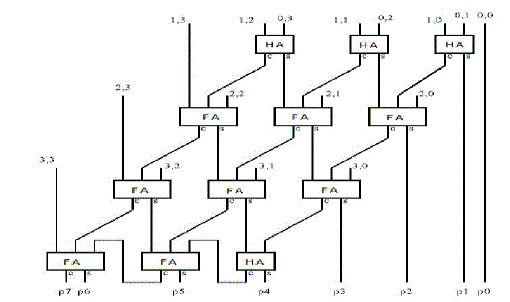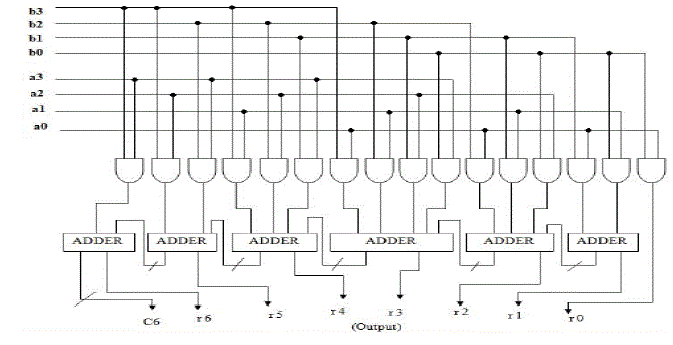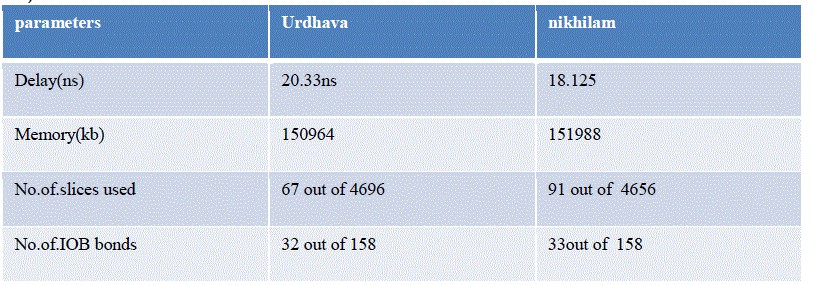ISSN ONLINE(2320-9801) PRINT (2320-9798)

All submissions of the EM system will be redirected to Online Manuscript Submission System. Authors are requested to submit articles directly to Online Manuscript Submission System of respective journal.

# Vedic Multiplier in VLSI for High Speed Applications

 G.Ramachandran1, T.Muthumanickam2, P.M.Murali3, Sajith.S.Nair4, L.Vasnath5 Assistant Professor, Dept. of ECE., VMKV Engineering College, Vinayaka Missions University, Tamilnadu, India Professor, Dept. of ECE., VMKV Engineering College, Vinayaka Missions University, Tamilnadu, India Assistant Professor, Dept. of ECE., VMKV Engineering College, Vinayaka Missions University, Tamilnadu, India II year B.M.E, Dept. of BME., VMKV Engineering College, Vinayaka Missions University, Tamilnadu, India Associate Professor, Dept. of BME., TEJAA SHAKTHI INSTITUTE OF TECHNOLOGY FOR WOMEN, Anna University, Tamilnadu, India Related article at Pubmed, Scholar Google

Visit for more related articles at International Journal of Innovative Research in Computer and Communication Engineering

## Abstract

Today most of the processor requires very high speed of operation. Usually DSP processors are based on mathematical approaches. In that multiply-accumulate operation plays vital role. Compared to addition, multiplication process takes large amount of time thus reduces the speed of the processor, consumes some amount of power and area. In this paper we proposed two techniques to improve processor speed based on Vedic mathematics. In Vedic mathematics among 16 sutras, 2 sutras are applicable for multiplication. First method URDHAVA TRIYAKBHYAM sutra which is similar to array multiplication .When number of bits increases, gate delay and area increases slowly compared to other multiplier. So the advanced technique called NIKHILAM sutra is employed. These sutras are meant for faster mental calculation. Though faster when implemented in hardware, it consumes more power than the conventional ones. In this project both the techniques are compared and found that nikhilam is best. This project presents a technique to modify the architecture of the Vedic multiplier by using some existing methods in order increase the processor speed.

### Keywords

Array multiplier, DSP,VLSI

### I. INTRODUCTION

Multiplication is an important fundamental function in arithmetic operations. Multiplication based operations such as Multiply and Accumulate(MAC) and inner product are among some of the frequently used Computation- Intensive Arithmetic Functions(CIAF) currently implemented in many Digital Signal Processing (DSP) applications such as convolution, Fast Fourier Transform(FFT), filtering and in microprocessors in its arithmetic and logic unit .The performance of multiplication is crucial for multimedia applications such as 3D graphics and signal processing systems, which depend on the execution of large numbers of multiplications Since multiplication dominates the execution time of most DSP algorithms, so there is a need of high speed multiplier. Currently, multiplication time is still the dominant factor in determining the instruction cycle time of DSP chip.

### II. SYSTEM ANALYSIS

#### A. MULTIPLIERS

In microprocessors multiplication operation is performed in a variety of forms in hardware and software depending on the cost and transistor budget allocated for this particular operation. In the beginning stages of computer development of any complex operation was usually programmed in software or coded in the micro-code of the machine. Design developed for a multiplier which generates the product of two numbers using purely combinational logic Today it is more likely to find full hardware implementation of the multiplication in order to satisfy growing demand for speed and due to the decreasing cost of hardware. The execution time of most DSP algorithms is dependent on its multipliers, and hence need for high speed multiplier arises.

#### B. ARRAY MULTIPLIERS

Array multiplier is an efficient layout of a combinational multiplier. The two's complement multiplication is converted to an equivalent parallel array addition problem in which each partial product bit is the AND of a multiplier bit and a multiplicand bit, and the signs of all the partial product bits are positive.In array multiplier, consider two binary numbers A and B, of m and n bits. There are mn summands that are produced in parallel by a set of mn AND gates. n x n multiplier requires n (n-2) full adders, n half-adders and n2 AND gates. Also, in array multiplier worst case delay would be (2n+1) td.Array Multiplier gives more power consumption as well as optimum number of components required, but delay for this multiplier is larger. It also requires larger number of gates because of which area is also increased; due to this array multiplier is less economical Thus, it is a fast multiplier but hardware complexity is high.Figure 2 Array multiplier diagram

### III.VEDIC MULTIPLIER

The use of Vedic mathematics lies in the fact that it reduces the typical calculations in conventional mathematics to very simple ones. This is so because the Vedic formulae are claimed to be based on the natural principles on which the human mind works. Vedic Mathematics is a methodology of arithmetic rules that allow more efficient speed implementation. This is a very interesting field and presents some effective algorithms which can be applied to various branches of engineering such as computing.
Vedic mathematics is based on 16 Sutras (or aphorisms) dealing with various branches of mathematics like arithmetic, algebra, geometry etc.These sutras are meant for faster mental calculation .These Sutras along with their brief meanings are enlisted below alphabetically.
These methods and ideas can be directly applied to trigonometry, plain and spherical geometry, conics, calculus (both differential and integral) and applied mathematics of various kinds.
Sri BharatiKrisnaTirtha.The implementation of vedic sutras ensure substantial reduction of propagation delay in comparison with Wallace Tree (WTM), modified Booth Algorithm (MBA), Baugh Wooley (BWM) and Row Bypassing and Parallel Architecture (RBPA) based implementation which are most commonly used architectures.

### A. DESCRIPTION OF URDHVA TIRYAKBHYAM

Line diagram for the multiplication and the digits on the both sides of the line are multiplied and added with the carry from the previous step. This generates one of the bits of the result and a carry. This carry is added in the next step and hence the processgoes on. If more than one line is there in one step, all the results are added to the previous carry. In each step, LSB acts as the result bit and all other bits act as carry for the next step. Initially the carry is taken to be zero. This process is repeated from LSB to MSB
B.HARDWARE ARCHITECTURE OF URDHAVA TRYAKBHYAMFigure 4 Hardware architecture of Urdhava Tiryakbhyam
Firstly, least significant bits are multiplied which gives the least significant bit of the product(vertical).Then, the LSB of the multiplicand is multiplied with the next higher bit of the multiplier and added with the product of LSB multiplier and next higher bit of the multiplicand(crosswise).The sum gives the second bit of the product and the carry is added in the output of next stage sum obtained by the crosswise and vertical multiplication and addition of three bits of the two numbers from least significant position.Next all the four bits are processed with crosswise multiplication and addition to give the sum and carry. The sum is the corresponding bit of the product and the carry is again added to the next stage multiplication and addition of three bits except the LSB.The same operation continues until the multiplication of the two MSBs to give the MSB of the product.

### V. RESULT ANALYSIS

A) RESULTTable 5. Result
In proposed method, the algorithm for nikhilam sutra has written based on mathematical operation so the delay for nikhilam sutra is reduced. Compared to existing method, the delay and memory for both the sutras are reduced.

### A.CONCLUSION

The performance of nikhilam sutra and urdhavatriyagbhyam was investigated. The8X8-bit Vedic multiplier using nikhilam sutra implementation uses less numbers of adders compared to 8X8 Vedic multiplier using “urdhavatriyagbhyam”. Nikhilam sutra reduces the multiplication of two large numbers into the multiplication of two small numbers and concatenation. Hence, there is significant reduction in the delay time which leads to improvement of speed and also the memory occupied is also reduced when using Nikhilam sutra.

### B FUTURE ENCHANCEMENTS

Usually DSP processors are based on mathematical approaches. In that multiply-accumulate operation plays vital role. Compared to addition, multiplication process takes large amount of time thus reduces the speed of the processor.In this project,multiplicationtechnique by using nikhilam sutra and urdhavatriyagbhyam sutras has been implemented. After comparing the simulation results, it is found that Nikhilam sutra is best for high speed applications. In this project delay is reduced around 35% in Nikhilam sutra and it can be further reduced around 50% by modifying the architecture in future. But the only drawback is power consumption is more.

### References

1. Esence of Vedas, Kapil Dev Dwivedi, Katyayan Vedic SahityaPrakashan, Hoshiarpur, 1990.
2. Vedic Concepts, Prof. B BChaubey, Katyayan Vedic SahityaPrakashan, Hoshiarpur, 1990.
3. APPLICATIONS OF THE VEDIC MATHEMATICS SUTRA Published by Inspiration Books, 2010,Kensglen, Nr Carsphairn, Castle Douglas, DG7 3TE, Scotland, U.K.
4. Asmita Haveliya”A Novel Design for High Speed Multiplier for Digital Signal Processing Applications” International Journal of Technology And Engineering System(IJTES)
5. Amit Gupta”Design of Fast,Low Power 16-bit Multiplier using Vedic Mathematics: A Modular Approach”LAP